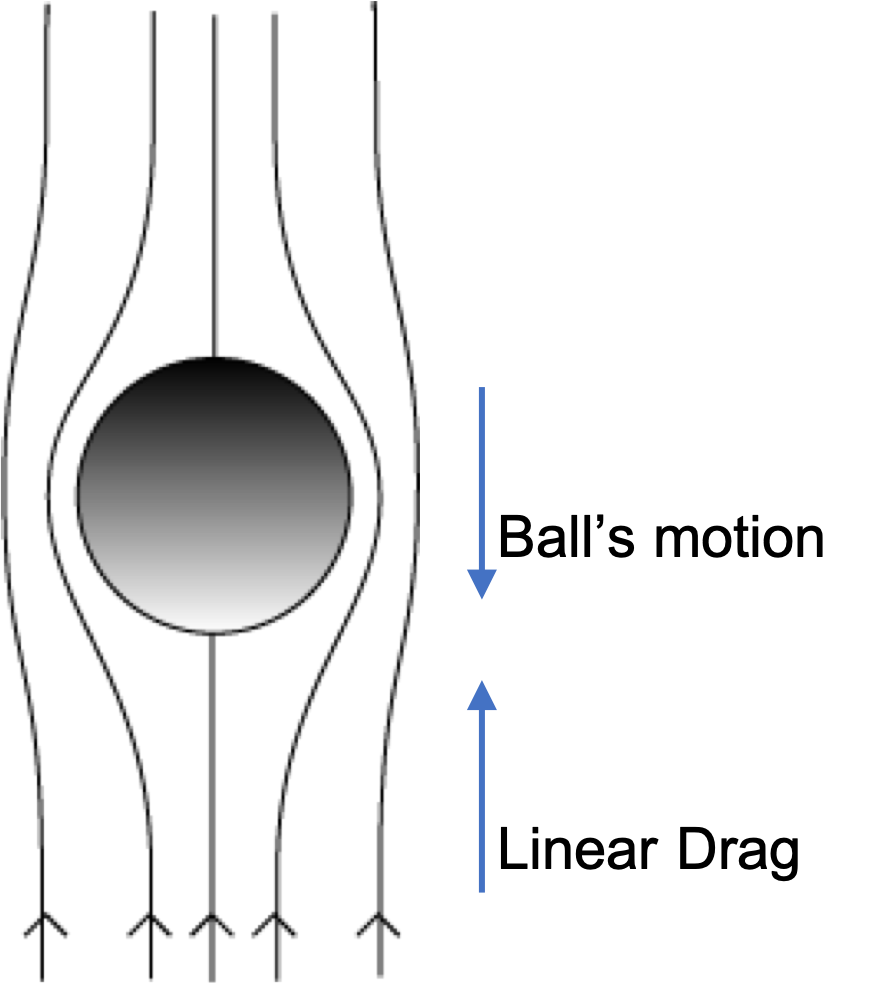## Section18.5Stoke's Law

The expression for the viscous drag force on a gently falling sphere in a fluid was deduced by Sir George Scott and is called the Stoke's law. This law finds use in many areas of physics and engineering.

Consider dropping a spherical ball in a still fluid, which can be liquid or gas. As the ball falls, it experiences a viscous force $\vec F_{\text{visc}}$ due to the laminar flow of fluid around the falling ball, depending upon the viscosity of the fluid. Let $R$ be the radius of the ball, $\vec v$ its velocity, and $\eta$ the viscosity of fluid. Stokes's law states the following expression for the viscous force $\vec F_{\text{visc}}\text{.}$

\begin{equation} \vec F_{\text{visc}} = -6\pi\eta R \vec v\tag{18.5.1} \end{equation}Note that the viscous force by Stokes's law is directly proportional to the radius rather than the area of cross-section as one might expect. Except for the factor $6 \pi\text{,}$ you can guess the formula by a dimensional analysis.

The argument for a dimensional analysis goes as follows. We assume that the magnitude of the viscous force will depend on the velocity, $F_{\text{visc}} \sim v^{m}\text{,}$ for some power $m$ to be determined by the dimensional argument here. We also know that it will involve the size of the ball, although we don't know if it will be $R$ or $R^2\text{,}$ or some other power of $R \text{,}$ say $R^n \text{.}$ The viscous force should also be proportional to the viscosity coefficient, $\eta\text{.}$ Writing out the dimension of the product of these dependencies of the viscous force, we find

\begin{equation*} [\eta][v^m][R^n] = \dfrac{[M][L]^{n+m-1}}{[T]^{m+1}}, \end{equation*}

which should equal the dimension of force, $\dfrac{[M][L]}{[T]^2}\text{.}$ Therefore, $m=1$ and $n=1\text{.}$ The dimensional analysis helps us guess the form of the magnitude of the viscous force on a spherical particle of radius R up to an undetermined constant $C\text{.}$

\begin{equation*} F_{\text{visc}} = C \eta R v. \end{equation*}

The calculations that shows that the constant $C = 6\pi$ is beyond this textbook. The direction of the viscous force is opposite to the direction of the velocity of the ball since it is a resistive force for the motion of the ball.

A steel ball of radius $2\text{ mm}$ is dropped gently in a cylindrical vessel filled with glycerin at $25^{\circ}\text{C}\text{.}$ At that temperature, the viscosity of glycerin is $0.95 \text{Pa.s}\text{.}$ The density of glycerin is $1260\text{ kg/m}^3$ and the density of steel is $8000\text{ kg/m}^3\text{.}$ After some time, the ball falls at constant speed.

(a) Ignoring the effect of buoyancy, find the terminal speed.

(b) What would be the terminal speed if you were to include the effect of buoyancy?

Hint

(a) Use balance of forces ignoring buoyancy. (b) Do same, now including buoyancy.

(a) $7.34\text{ cm/s} \text{,}$ (b) $6.19\text{ cm/s} \text{.}$

Solution 1 (a)

(a) At terminal speed all forces on the ball are balanced. Ignoring buoyancy leaves only weight and the viscous drag forces on the ball.

\begin{equation*} 6\pi\eta R v = mg, \end{equation*}

where

\begin{equation*} m = V\rho = \dfrac{4}{3}\pi R^3 \rho. \end{equation*}

Therefore,

\begin{equation*} v = \dfrac{2}{9}\, \dfrac{R^2 \rho g}{\eta}. \end{equation*}

Putting in the numerical values, I got

\begin{equation*} v = 0.0734\text{ m/s} = 7.34\text{ cm/s}. \end{equation*}
Solution 2 (b)

If we wish to include buoyancy, the equation is 6\pi\eta R v = mg - m_0 g,

where $m_0$ is the mass of glycerin displaced.
\begin{equation*} m_0 = V\rho_0 = \dfrac{4}{3}\pi R^3 \rho_0. \end{equation*}
Therefore,
\begin{equation*} v = \dfrac{2}{9}\, \dfrac{R^2 (\rho-\rho_0) g}{\eta} = 0.0619\text{ m/s}. \end{equation*}

A spherical ball of radius R and density $\rho$ is dropped from rest in a fluid of density $\rho_0\text{.}$ (a) Derive a formula for the terminal speed. (b) Derive a formula for the time it takes to reach the terminal speed.

Hint

(a) $\dfrac{g}{\gamma}\text{,}$ (b) $\infty\text{.}$

Solution 1 (a)

Let us orient the $y$-axis vertically down and write the $y$-component of the equation of motion of the ball.

\begin{equation} m\dfrac{d^2y}{dt^2} = mg - b \dfrac{dy}{dt}, \label{eq-ch13-f-d-ex-11-1}\tag{18.5.2} \end{equation}

where

\begin{equation*} b = 6\pi \eta R. \end{equation*}

Divide both sides of Eq. (18.5.2) by $m$ and write $\gamma$ for $b/m\text{,}$

\begin{equation*} \gamma \equiv \dfrac{b}{m}, \end{equation*}

to obtain

\begin{equation} \dfrac{d^2y}{dt^2} + \gamma \dfrac{dy}{dt} = g. \label{eq-ch13-f-d-ex-11-2}\tag{18.5.3} \end{equation}

This equation is not that hard to solve, but I will not go through the steps of the solution. I will give you the answer instead. You will find that for $y(0) = 0$ and $v_y(0) = \dfrac{dy}{dt}(0) = 0$ the $y$-coordinate of the center of the ball will turn out to be

\begin{equation*} y(t) = \dfrac{g}{\gamma^2} \left[ e^{-\gamma t} + \gamma t - 1 \right]. \end{equation*}

You can convince yourself that this the solution of Eq. (18.5.3) by plugging this function in that equation. Furthermore, you can verify that that this solution satisfies the initial conditions as well. Now, this solution should tell us when the terminal speed will be reached.

We get velociy at $t$ by taking a derivative of $y\text{.}$

\begin{equation*} v_y = \dfrac{dy}{dt} = \dfrac{g}{\gamma}\left( 1 -e^{-\gamma t} \right). \end{equation*}

Taking $t\rightarrow 0$ limit gives the final velocity to be

\begin{equation*} v_\text{terminal} = \dfrac{g}{\gamma}. \end{equation*}
Solution 2 (b)

(b) At the terminal speed the acceleration will be zero. Therefore we set the acceleration at the instant $t=T$ to zero and solve for $T\text{.}$

\begin{equation*} \dfrac{d^2y}{dt^2} = g e^{-\gamma T} = 0, \end{equation*}

which says that the acceleration actually becomes zero only asymptotically. The math answer will be $T=\infty\text{.}$ But, if we are willing to accept that if the acceleration is below some value, say $\epsilon g\text{,}$ where we set some value of $\epsilon$ such as $1/1000$ or so, then we can get a definite value for $T\text{.}$

\begin{equation*} e^{-\gamma T} = \epsilon\ \ \Longrightarrow\ \ T = -\dfrac{\ln{(\epsilon)}}{\gamma}. \end{equation*}